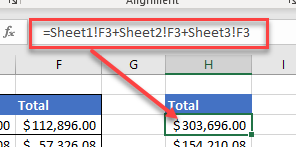# VBA Evaluate Function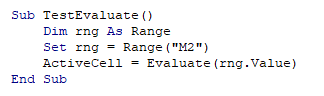The Evaluate Function in Excel is a little known function that can be extremely useful when writing VBA code.

## Evaluate Syntax

The function takes one argument, and converts what is held in that argument to an object or a value.

``Evaluate (Name)``

The name can be the name of an object (for example a cell address) or a formula. The Name cannot be longer than 255 characters.

There are a number of ways to use this function.

## Return a Result to Excel with Evaluate

Let us consider the following values in cell B2.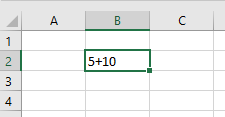If we want to add the values together, we could start with an equals (=) sign in cell B2 turning the values into a formula, and then get the value of 15.

However, in VBA code, using the Evaluate function, we can return the value 15 to the spreadsheet without entering a formula into cell B2.

``````Sub TestEvaluate()
Dim rng As Range
Set rng = Range("B2")
ActiveCell = Evaluate(rng.Value)
End Sub``````

First, we declare a range object and then populate that range object with the cell address B2.

We then use the Evaluate function to evaluate the value of the Range object and return the value to a different cell, in this case, C2.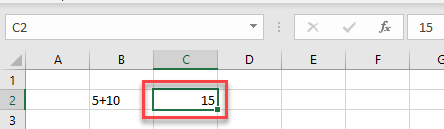As we can see if we debug the code, the immediate window will give us the value returned by the Evaluate function.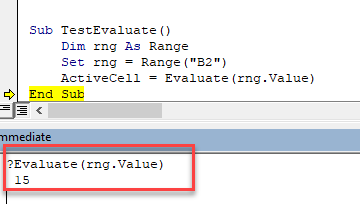## Format a Cell with Evaluate

We can also use the Evaluate function to format a cell.

``````Sub TestEvaluateFormat()
Dim strBld As String
Worksheets("Sheet3").Activate
strBld = "C2"
Application.Evaluate(strBld).Font.Bold = True
End Sub``````

This time we are passing a cell reference to a string variable, and then using that string variable in the Name argument of the Evaluate function to set the Bold property of the cell to true.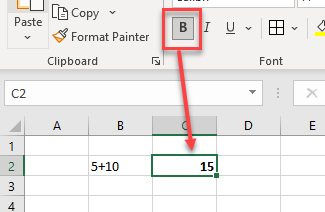## Shorten Formulas with the Evaluate Function

The Evaluate function can be used to shorten formulas in VBA code.

Let us look at the following code example:

``````Sub GetTotals()
Dim dblSales As Double
Dim dblExpenses As Double
Dim dblProfit As Double

dblSales = Worksheets("Sheet1").Range("F3") + Worksheets("Sheet2").Range("F3") + Worksheets("Sheet3").Range("F3")
dblExpenses = Worksheets("Sheet1").Range("F4") + Worksheets("Sheet2").Range("F4") + Worksheets("Sheet3").Range("F4")
dblProfit = dblSales - dblExpenses
End Sub``````

Using the Evaluate function, we can re-write the code as shown below:

``````Sub GetTotalsEvaluate()
Dim dblSales As Double
Dim dblExpenses As Double
Dim dblProfit As Double

dblSales = Evaluate("Sheet1!F3+Sheet2!F3+Sheet3!F3")
dblExpenses = Evaluate("Sheet1!F4+Sheet2!F4+Sheet3!F4")
dblProfit = dblSales - dblExpenses
End Sub``````

The Evaluate object removes the need to use the Worksheet or Range object individually as we can write this in a text string.  The format in this text string is identical to the format you would have used in the function bar in Excel if you were to create the formula there.